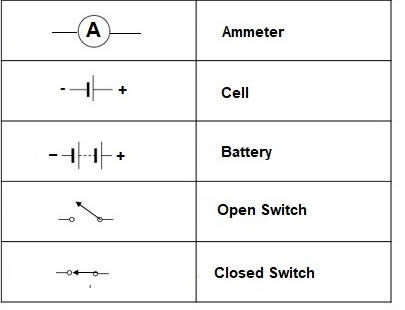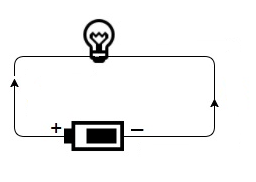# Grade 7, Science Olympiad (CBSE) - Electric circuit and symbols for electrical components

## Grade 7  |   Science  |   Electric circuit and symbols for electrical components, Electric current and its effects, Olympiad, CBSE, ICSE, SOF, ITO

`Electric circuit`

An electric current is a flow of particles (electrons) flowing through wires and components. It is the rate of flow of charge. If the electric charge flows through a conductor, we say that there is an electric current in the conductor. In the circuits using metallic wires, electrons constitute a flow of charges.

The schematic diagram represents the different components of a circuit; this is the circuit diagram. These symbols represent the common electrical components.The picture below shows a closed circuit diagram.